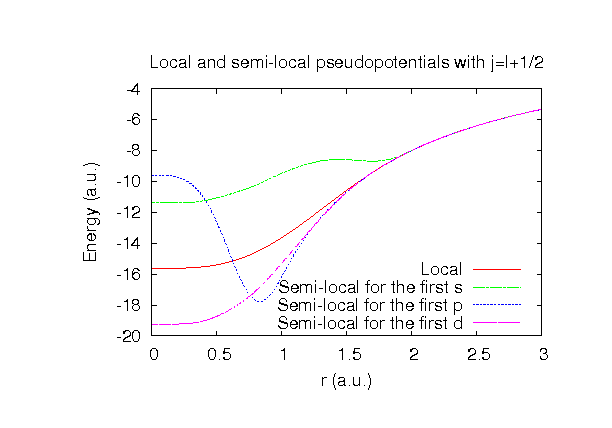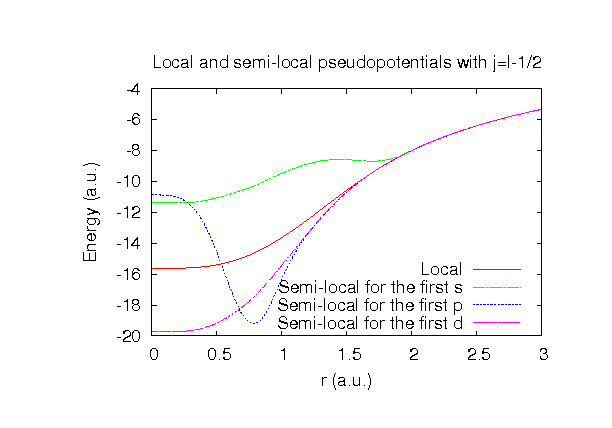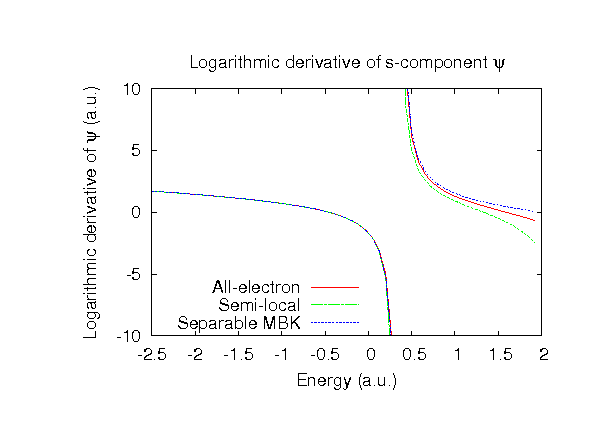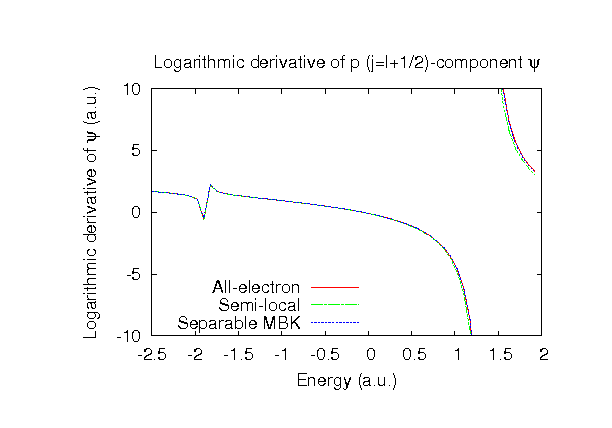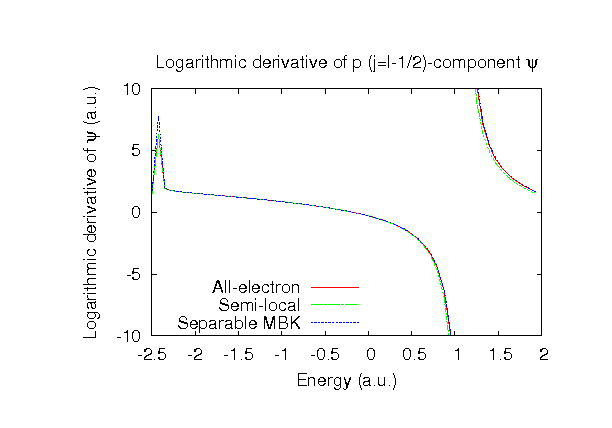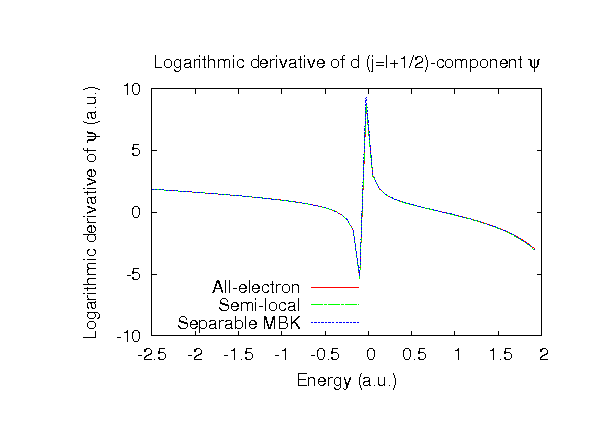### Fully relativistic pseudopotentials

Fully relativistic pseudopotentials generated by the MBK (PRB 47, 6728 (1993)) scheme within LDA (CA13) and GGA (PBE13) which contain a partial core correction and fully relativistic effects including spin-orbit coupling.

### Pseudo-atomic orbitals

The number below the symbol means a cutoff radius (a.u.) of the confinement potential. These file includes fifteen radial parts for each angular momentum quantum number l (=0,1,2,3,4). The basis functions were generated by variationally optimizing the corresponding primitive basis functions in the single atom, the dimer molecule, and the FCC bulk. The input files used for the orbital optimization can be found at Pt_opt.dat , Pt2_opt.dat , and Ptfcc_opt.dat . Since Pt_CA13.vps and Pt_PBE13.vps include the 5p, 5d, and 6s (16 electrons) as the valence states, the minimal basis set is Pt*.*-s1p1d1. Our recommendation for the choice of cutoff radius of basis functions is that Pt7.0.pao is enough for bulks, but Pt9.0.pao or Pt11.0.pao is preferable for molecular systems.

### Benchmark calculations by the PBE13 pseudopotential with the various basis functions

(1) Calculation of the total energy as a function of lattice constant in the fcc structure, where the total energy is plotted relative to the minimum energy for each case. a0 and B0 are the equilibrium lattice constant and bulk modulus obtained by fitting to the Murnaghan equation of state. The difference between Pt7.0-s2p2d2f1 and Pt7.0-s4p3d2f2 in the total energy at the minimum point is 0.0074 eV/atom. An input file used for the OpenMX calculations can be found at Ptfcc-EvsV.dat . For comparison the result by the Wien2k code is also shown, where the calculation was performed by default setting in the Ver. 9.1 of Wien2k except for the use of RMT x KMAX of 12.(2) Calculations of the band dispersion in the fcc structure, where the non-spin polarized collinear calculation with the lattice constant of 3.92 Ang. was performed using Pt_PBE13.vps and Pt7.0-s2p2d2f1, and the origin of the energy is taken to be the Fermi level. The input file used for the OpenMX calculations can be found at Ptfcc-Band.dat . For comparison the result by the Wien2k code is also shown, where the calculation was performed by default setting in the Ver. 9.1 of Wien2k except for the use of RMT x KMAX of 12.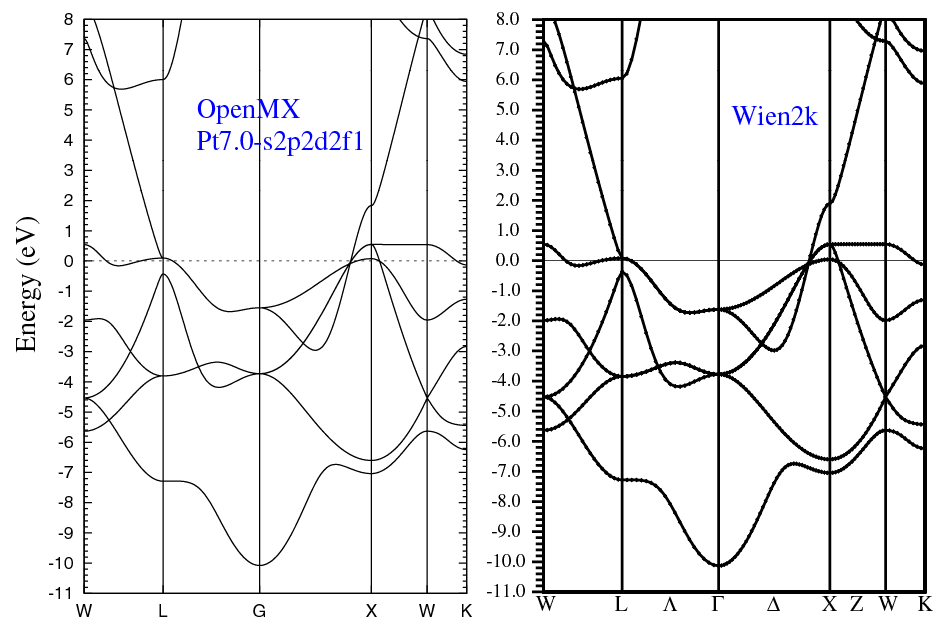(3) Calculations of the Pt2 molecule with S=3, where Pt_PBE13.vps and Pt9.0-s2p2d2f1 or Pt9.0-s4p3d2f2 were used. The input files used for the OpenMX calculations can be found at Pt2.dat , Pt.dat , and Pt_CP.dat .

 Equilibrium bond length (Ang.) Atomization energy (eV) Atomization energy (couterpoise corrected) (eV) Pt9.0-s2p2d2f1 2.394 3.233 3.230 Pt9.0-s4p3d2f2 2.378 3.344 3.340 Other calc. 2.339 a 3.33 a Expt. 2.333 b 3.14 b

a PW91 result: J. Anton et al., Phys. Rev. Lett. 89, 213001 (2002).
b M.M. Airola and M.D. Morse, J. Chem. Phys. 116, 1313 (2002).

### Supplementary information for the GGA (PBE13) pseudopotential